×

Search anything:

# Vector in C++

#### C++ vector Software EngineeringGet this book -> Problems on Array: For Interviews and Competitive Programming

Vector is a sequence container that can store elements, but its size can change dynamically (C/C++ array’s size do not change dynamically). Container is a objects that hold data of same type. Sequence containers store elements strictly in linear sequence. We can store int, string, float elements, depending on how we initialize the vector.

For example:
Below is definition of std::vector from `vector` header file

``````vector<int> v;
``````

Unlike array, vector can shrink or expand as needed at run time. The storage of the vector is handled automatically. To support shrink and expand functionality at runtime, vector container may allocate some extra storage to accommodate for possible growth thus container have actual capacity greater than the size. Therefore, compared to array, vector consumes more memory in exchange for the ability to manage storage and grow dynamically in an efficient way.

Certain functions associated with the vector are:

### Iterators

• begin() – Returns an iterator pointing to the first element in the vector
• end() – Returns an iterator pointing to the theoretical element that follows the last element in the vector
• rbegin() – Returns a reverse iterator pointing to the last element in the vector (reverse beginning). It moves from last to first element
• rend() – Returns a reverse iterator pointing to the theoretical element preceding the first element in the vector (considered as reverse end)
• cbegin() – Returns a constant iterator pointing to the first element in the vector.
• cend() – Returns a constant iterator pointing to the theoretical element that follows the last element in the vector.

### Capacity

• size() – Returns the number of elements in the vector.
• max_size() – Returns the maximum number of elements that the vector can hold.
• capacity() – Returns the size of the storage space currently allocated to the vector expressed as number of elements.
• resize() – Resizes the container so that it contains ‘g’ elements.
• empty() – Returns whether the container is empty.
• shrink_to_fit() – Reduces the capacity of the container to fit its size and destroys all elements beyond the capacity.
• reserve() – Requests that the vector capacity be at least enough to contain n elements.

### Modifiers

• assign() – It assigns new value to the vector elements by replacing old ones
• push_back() – It push the elements into a vector from the back
• pop_back() – It is used to pop or remove elements from a vector from the back.
• insert() – It inserts new elements before the element at the specified position
• erase() – It is used to remove elements from a container from the specified position or range.
• swap() – It is used to swap the contents of one vector with another vector of same type. Sizes may differ.
• clear() – It is used to remove all the elements of the vector container

### Some basic functionalities

• We have stored 5 integers in our vector:
``````vector<int> v;
v.push_back(2);
v.push_back(5);
v.push_back(1);
v.push_back(3);
v.push_back(4);
``````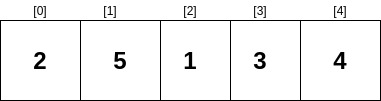• If we use pop_back(), we’ll remove the last element.
``````v.pop_back();
``````

Now, only 4 elements will and the last element will be popped out, i.e.,

``````2 5 1 3
``````

Now, we have only 4 elements,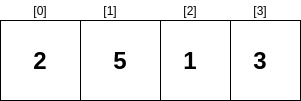• To remove the first element, we can use erase(). We need to pass the element’s position (iterator position), we want to remove, as an argument.
``````v.erase(v.begin());
``````

Now, there are only 4 elements in the vector v and first element i.e., 2 is erased.

``````5 1 3 4
``````

Elements left are,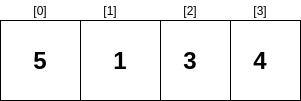• We can also remove the last element using erase.
``````v.erase(v.begin() + v.size() - 1);
``````

Now, we haveSince erasing an element requires moving other elements (to ensure random access), time complexity of erase is O(1).

• To get the first element, we use front,
``````v.front();
``````

We will get the first element, i.e.,

``````2
``````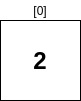• To the last element, we use back
``````v.back();
``````

We get the last element, i.e.,

``````4
``````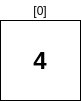• To the know the number of element inside the vector, we use size
``````v.size();
``````

We will get to know the number of vector v;

``````5
``````

Instead of using size() we can use empty() method.

``````v.empty();
``````

### size() v/s empty()

empty() function is often said to be preferred over the size() function due to some of these points-

• empty() function does not use any comparison operators, thus it is more convenient to use.

• empty() function is implemented in constant time, regardless of container type, whereas some implementations of size() function require O(n) time complexity such as list::size().

• As a simple array, we can use [] and = operators

``````v = 10;
v = 20;
v = 30;
cout << v << endl; // 10
cout << v << endl; // 20
cout << v << endl; // 30
``````

Output

``````10
20
30
``````
• To remove all the elements from the vector, we use,
``````v.clear();
``````

## Using algorithm

We can use algorithm's sort to order the vector elements in an ascending order.

``````#include <algorithm>
sort(v.begin(), v.end());
``````

The vector will get sorted in ascending order, like;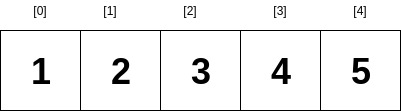And in a descending order, using the greater< int> comparison as the third argument.

``````#include <algorithm>
sort(v.begin(), v.end(), greater<int>());
``````

This is what we will get,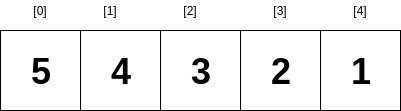In order to avoid using `sort(v.begin(), v.end(), greater<int>());`, we can put this code into a void function, passing the vector as an argument.

This can be done in 2 ways:

1.As a reference

``````void desc_sort(vector<int> &v) {
sort(v.begin(), v.end(), greater<int>());
}

desc_sort(v);
``````

2.As a pointer

``````void desc_sort(vector<int> *v) {
sort(v->begin(), v->end(), greater<int>());
}

desc_sort(&v);
``````

In both of the above cases, we will get a vector sorted in descending order.#### Shreya Gupta

Software Development Lead at Developer Student Club Thapar | Intern at OpenGenus | B. Tech in Civil Engineering at Thapar Institute of Engineering and Technology